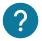# How to control a digital Pin? How to use digitalWrite and digitalRead?

The writing or Reading of a digital Pin will be used to control a component and read the value of a sensor.

Here is what we will see in this course:

• Setup the pin as an input or output (pinMode)
• Control a component (digitalWrite)

Here is the digital pin which can be controlled by digitalWrite and digitalRead:As you see there are some pins with a wave symbol. This pins can use the pwm (pulse width modulation). You can control them by two ways: with digitalWrite or digitalRead.

With the digitalWrite you can turn on a pin at 5V or turn off it. By using AnalogWrite, you choose choose exactly the tension to turn on a led at the half of it’s power for example.

A digital Pin without a wave symbol can be controlled just with digitalWrite.### What is the difference between Analog and digital?

A digital signal can have two states: HIGH and LOW. HIGH corresponds to 5V and LOW corresponds to 0V. This signal is really useful to detect the state of a push button, or to turn on a LED.

Nevertheless if you want to turn on a LED with the half of its power, you will nedd the analogic signal.

The analogic signal can have an infinity of value between 0V and 5V. This signal will be used to measure and sends many values more accurate, such as 0.45V, while a digital signal you can have only 0V or 5V.

## pinMode ()

PinMode is a function to defined if a pin is an input or output. In other words, if the arduino card has to wait some values from pin or give some values to it to control a component for example.

The pinMode has two parameters : the name of the pin you gave or tis number and the mode : INPUT or OUTPUT :

``pinMode(pin, mode);``

The pins, have we have seen on the Arduino card’s image, is from 0 to 13. Nevertheless, the pins 0 and 1 is used for specific cases.

Here is an example for the pin 10 as an output:

``pinMode(10, OUTPUT);``

Here is an example for the pin 5 as an input:

``pinMode(5, INPUT);``

## digitalWrite ()

If your pin has been configured as an output, you will need digitalWrite to control it. That way you can control a component. The function digitalWrite will give current to your component to turn it on (HIGH), or connect if to the ground to turn off it (LOW).

With the digitalWrite you have two parameters: the pin and the value (HIGH or LOW):

``digitalWrite(pin, value);``

With this example we can see that the function takes two parameters:

• pin: from 2 to 13
• value:  HIGH to have 5V and LOW for the 0V

Here is an example with the pin 7:

``digitalWrite(7, HIGH);``

This is an another example to turn off a component connected to the pin 10:

``digitalWrite(10, LOW);``

The digitalRead is used to read information in input of the Arduino card. It’s really useful for knowing if the user has pressed the push button for example.

``digitalRead(pin);``
``digitalRead(8);``